• 利用MATLAB指令录制一段语音信号，对其进行时域波形的观察频域的谱分析。根据该信号的频谱构成，选择三种不同的采样频率重新录制该语音信号，并试听回放效果，进行比较，以验证采样定理。
• 实验一 [y,fs,bit]=wavread'I do%读取音乐片段fs是采样率 size(y%求矩阵行数列数 y1=y,1%对信号进行分列处理 n1=length(y1%取y长度 t1=(0:n1-1)/fs%设置波形图横坐标 figure subplot(2,1,1; plot(t1,y1; %画出...
• 然后在Matlab软件平台下，利用函数audioread对语音信号进行采样，记住采样频率和采样点数，后利用函数FFT对信号进行快速傅里叶变换，得到信号的频谱特性，然后加入一固定频率干扰信号，再画出语音信号干扰前后的时域...
• PAGE PAGE 1 基于MATLAB语音信号的采集与分析 我们通过学习使用MATLAB仿真软件实现语音信号分析加深对信号与系统这门课程所学习内容的理解锻炼自学能力动手能力我们通过电脑的声卡采集声音信号借助已有的知识...
• 通过PC机录制自己的一段声音，运用Matlab提供的函数进行仿真分析，并画出采样语音信号的时域波形和频谱图，对所采集的语音信号加入干扰随机高斯噪声，对加入噪声的信号进行播放，并进行时域和频谱分析；...
• 1.语音信号采集：录音几秒钟，采样频率20000多，任何格式都可以。 2.加噪声不能有用信号混叠，可以是单频噪声也可以是多频噪声。音乐信号语音采集时候加频谱分析。 3.进行频谱分析时，频谱图横坐标要单位Hz...
• b=menu('请选择：','原始信号采样时域图和频谱图','FIR滤波器','IIR滤波器','退出'); if b==1 for j=1:3 temp=menu('请选择','播放原始语音','原始语音时域图和频谱图','FFT频谱图'); if temp==1 ...
for i=1:3
b=menu('请选择：','原始信号采样后的时域图和频谱图','FIR滤波器','IIR滤波器','退出');
if b==1
for j=1:3
temp=menu('请选择','播放原始语音','原始语音时域图和频谱图','FFT频谱图');
if temp==1
clc;
clear;
[x,fs]=audioread('D:\matlab\bin\张婷婷.wav'); %打开语音信号
sound(x,fs); %播放语音信号
%main(1)
else
if temp==2
[x,fs]=audioread('D:\matlab\bin\张婷婷.wav'); %打开语音信号
sound(x,fs); %播放语音信号
N=length(x); %长度
n=0:N-1;
w=2*n*pi/N;
y1=fft(x); %对原始信号做FT变换
subplot(2,1,1);
plot(n,x) %做原始语音信号的时域波形图
title('原始语音信号时域图');
xlabel('时间t');
ylabel('幅值');

subplot(2,1,2); %做原始语音信号的频谱图
plot(w/pi,abs(y1));
title('原始语音信号频谱')
xlabel('频率Hz');
ylabel('幅度');
%main(1);
else

[x,fs]=audioread('D:\matlab\bin\张婷婷.wav'); %打开语音信号sound(x,fs); %播放语音信号
N=length(x); %长度
n=0:N-1;
w=2*n*pi/N;
y1=fft(x); %对原始信号做FFT变换
subplot(2,1,1); %做原始语音信号的频谱图
plot(w/pi,abs(y1));
title('原始语音信号频谱')
xlabel('频率Hz');
ylabel('幅度');
df=fs/(N-1); %分辨率
f=(0:N-1)*df; %其中每点的频率
Y=fft(x(1:N))/N*2; %真实的幅值 %
Y=fftshift(Y);
subplot(2,1,2); %做原始语音信号的频谱图
plot(f(1:N/2),abs(Y(1:N/2)));
title('原始语音信号采样后的FFT频谱')
xlabel('频率Hz');
ylabel('幅值');

end
end
end
else
if b==2
for k=1:3
temp1=menu('请选择','FIR低通滤波器','FIR高通滤波器','FIR带通滤波器');
if temp1==1
%FIR低通
[x,fs]=audioread('D:\matlab\bin\张婷婷.wav'); %打开语音信号
sound(x,fs);  %播放滤波后的语音信号

wp=2*pi*1000/fs;
ws=2*pi*1200/fs;
Rp=1;
Rs=53;
wdelta=ws-wp;
N=ceil(8* pi/wdelta);  %取整
wn=(wp+ws)/2;
[b,a]=fir1(N,wn/pi,hamming(N+1));  %选择窗函数，并归一化截止频率

figure(1)
freqz(b,a,512);
title('FIR低通滤波器');
f2=filter(b,a,x);

figure(2)
subplot(2,2,1)
plot(x)
title('FIR低通滤波器滤波前的时域波形');

subplot(2,2,2)
plot(f2);
title('FIR低通滤波器滤波后的时域波形');
pause(2);
sound(f2,fs);  %播放滤波后的语音信号

F0=fft(f2,1024);
f=fs*(0:511)/1024;

y2=fft(x,1024);
subplot(2,2,3);
plot(f,abs(y2(1:512)));
title('FIR低通滤波器滤波前的频谱')
xlabel('频率/Hz');
ylabel('幅值');

subplot(2,2,4)
F2=plot(f,abs(F0(1:512)));
title('FIR低通滤波器滤波后的频谱')
xlabel('频率/Hz');
ylabel('幅值');
ylabel('幅值');xlabel('频率/Hz');
ylabel('幅值');
%main(2);
else
if temp1==2
%FIR高通
[x,fs]=audioread('D:\matlab\bin\张婷婷.wav'); %打开语音信号
sound(x,fs);
wp=2*pi*5000/fs;
ws=2*pi*3600/fs;
Rp=1;
Rs=53;
wdelta=wp-ws;
N=ceil(8*pi/wdelta);  %取整
wn=(wp+ws)/2;
[b,a]=fir1(N,wn/pi,'high');

figure(1)
freqz(b,a,512);
title('FIR高通滤波器');

f2=filter(b,a,x);
figure(2)

subplot(2,2,1)
plot(x)
title('FIR高通滤波器滤波前的时域波形');

subplot(2,2,2)
plot(f2);
title('FIR高通滤波器滤波后的时域波形');
pause(2);
sound(f2,fs);  %播放滤波后的语音信号

F0=fft(f2,1024);
f=fs*(0:511)/1024;

y2=fft(x,1024);
subplot(2,2,3);
plot(f,abs(y2(1:512)));
title('FIR高通滤波器滤波前的频谱')
xlabel('频率/Hz');
ylabel('幅值');

subplot(2,2,4)
F2=plot(f,abs(F0(1:512)));
title('FIR高通滤波器滤波后的频谱')
xlabel('频率/Hz');
ylabel('幅值');
% main(2);
else

%FIR 带通
[x,fs]=audioread('D:\matlab\bin\张婷婷.wav'); %打开语音信号
sound(x,fs);
wp1 =2*pi*1400/fs;wp2=2*pi*3400/fs;
ws1 =2*pi*1200/fs;ws2=2*pi*3400/fs;
Rp=1;
Rs=53;
wp=(wp1 +ws1)/2;ws=(wp2+ws2)/2;
wdelta=wp1-ws1;
N=ceil(8*pi/wdelta);  %取整
wn=[wp ws];
[b,a]=fir1(N,wn/pi,'bandpas');

figure(1)
freqz(b,a,512);
title('FIR带通滤波器');

f2=filter(b,a,x);
figure(2)

subplot(2,2,1)
plot(x)
title('FIR带通滤波器滤波前的时域波形');

subplot(2,2,2)
plot(f2);
title('FIR带通滤波器滤波后的时域波形');
pause(2);
sound(f2,fs);  %播放滤波后的语音信号

F0=fft(f2,1024);
f=fs*(0:511)/1024;

y2=fft(x,1024);

subplot(2,2,3);
plot(f,abs(y2(1:512)));
title('FIR带通滤波器滤波前的频谱')
xlabel('频率/Hz');
ylabel('幅值');

subplot(2,2,4)
plot(f,abs(F0(1:512)));
title('FIR带通滤波器滤波后的频谱')
xlabel('频率/Hz');
ylabel('幅值');
%main(2)
end
end
end
else
for l=1:3
temp2=menu('请选择','IIR低通滤波器','IIR高通滤波器','IIR带通滤波器');
if temp2==1
%IIR低通
[x,fs]=audioread('D:\matlab\bin\张婷婷.wav'); %打开语音信号
sound(x ,fs);

Ts=1/fs;R1=100;
wp=2*pi*1000/fs;
ws=2*pi*1200/fs;
Rp=1;
Rl=8;
wp1=2/Ts*tan(wp/2);  %将模拟指标转换成数字指标
ws1=2/Ts*tan(ws/2);
[N,Wn]=buttord(wp1,ws1,Rp,Rl,'s'); %选择滤波器的最小阶数
[Z,P,K]=buttap(N);   %创建butterworth模拟滤波器
[Bap,Aap]=zp2tf(Z,P,K);
[b,a]=lp2lp(Bap,Aap,Wn);
[bz,az]=bilinear(b,a,fs);  %用双线性变换法实现模拟滤波器到数字滤波器的转换

[H,W]=freqz(bz,az);  %绘制频率响应曲线
figure(1)
plot(W*fs/(2* pi),abs(H))
grid
xlabel('频率/ Hz')
ylabel('频率响应幅度')
title('IIR低通滤波器')

figure(2)
subplot(2,2,1)
plot(x)  %画出滤波前的时域图
title('IIR低通滤波器滤波前的时域波形');

y1=filter(bz,az,x);
subplot(2,2,2)
plot(y1);  %画出滤波后的时域图
title('IIR低通滤波器滤波后的时域波形');
pause(2);
sound(y1 ,41000);  %播放滤波后的信号

F0=fft(y1,1024);
f=fs*(0:511)/1024;

y2=fft(x,1024);
subplot(2,2,3);
plot(f,abs(y2(1:512)));  %画出滤波前的频谱图
title('IIR低通滤波器滤波前的频谱')
xlabel('频率/Hz');
ylabel('幅值');

subplot(2,2,4)
F1=plot(f,abs(F0(1:512))); %画{出滤波后的频谱图
title('lIR低通滤波器滤波后的频谱')
%main(3);
else
if temp2==2
%IIR高通
[x,fs]=audioread('D:\matlab\bin\张婷婷.wav'); %打开语音信号
sound(x,fs);
Ts=1/fs;R1=50;
Wp=2*pi*4400/fs;
Ws=2* pi*4000/fs;
Rp=1;
Rl=100;
Wp1=2/Ts*tan(Wp/2);  %将模拟指标转换成数字指标
Ws1=2/Ts*tan(Ws/2);

[N,Wn]=cheb2ord(Wp1,Ws1,Rp,R1,'s'); %选择滤波器的最小阶数
[Z,P,K]=cheb2ap(N,Rl);  %创建切比雪夫模拟滤波器
[Bap,Aap]=zp2tf(Z,P,K);
[b,a]=lp2hp(Bap,Aap,Wn);
[bz,az ]=bilinear(b,a,fs);  %用双线性变换法实现模拟滤波器到数字滤波器的转换

[H,W]=freqz(bz,az);  %绘制频率响应曲线
figure(1)
plot(W*fs/(2*pi),abs(H))
grid
xlabel('频率/ Hz')
ylabel('频率响应幅度')
title('IIR高通滤波器')

f1=filter(bz,az,x);
figure(2)

subplot(2,2,1)
plot(x)  %画出滤波前的时域图
title('IIR高通滤波器滤波前的时域波形');

subplot(2,2,2)
plot(f1);  %画出滤波后的时域图
title('IIR高通滤波器滤波后的时域波形');
pause(2);
sound(f1,44100);  %播放滤波后的信号

F0=fft(f1,1024);
f=fs*(0:511)/1024;

y2=fft(x,1024);
subplot(2,2,3)
plot(f,abs(y2(1:512)));  %画出滤波前的频谱图
title('IIR高通滤波器滤波前的频谱')
xlabel('频率/Hz');
ylabel('幅值');

subplot(2,2,4);
plot(f,abs(F0(1:512)));  %画出滤波后的频谱图
title('IIR高通滤波器滤波后的频谱')
xlabel('频率/Hz');
ylabel('幅值');
%main(3);
else
%IIR带通
[x,fs]=audioread('D:\matlab\bin\张婷婷.wav'); %打开语音信号
sound(x,fs);
Ts= 1/fs;R1=30;
fb1=1200;fb2=3000;fc1=1000;fc2=3200;fs=23000;
W1=2*fb1 *pi/fs;W2=2*fc1*pi/fs;
W3=2*fb2*pi/fs;W4=2*fc2* pi/fs;
Wp=[W1,W3];
Ws=[W2,W4];
Rp=1;
Rl=100;
Wp1=2/Ts*tan(Wp/2);  %将模拟指标转换成数字指标
Ws1 =2/Ts*tan(Ws/2);

[N,Wn]=cheb2ord(Wp1,Ws1,Rp,R1,'s'); %选择滤波器的最小阶数
[Z,P,K]=cheb2ap(N,Rl);   %创建切比雪夫模拟滤波器;
[Bap,Aap]=zp2tf(Z,P,K);
[b,a]=lp2bp(Bap,Aap,2100*2*pi,1800*2*pi);
[bz,az]=bilinear(b,a,fs);  %用双线性变换法实现模拟滤波器到数字滤波器的转换

[H,W]=freqz(bz,az);  %绘制频率响应曲线
figure(1)
plot(W*fs/(2*pi),abs(H))
grid
xlabel('频率/ Hz')
ylabel('频率响应幅度')
title('IIR带通滤波器')

f1 =filter(bz,az,x);
figure(2)
subplot(2,2,1)
plot(x)   %画出滤波前的时域图
title('IIR带通滤波器滤波前的时域波形');

subplot(2,2,2)
plot(f1);   %画出滤波后的时域图
title('IIR带通滤波器滤波后的时域波形');
fs2=50000;
pause(2);
sound(f1 ,fs2);  %播放滤波后的信号

F0=fft(f1,1024);
f=fs*(0:511)/1024;

y2=fft(x,1024);
subplot(2,2,3);
plot(f,abs(y2(1:512))); %画出滤波前的频谱图
title('IIR带通滤波器滤波前的频谱')
xlabel('频率/Hz');
ylabel('幅值');

subplot(2,2,4)
plot(f,abs(F0(1:512)));  %画出滤波后的频谱图
title('IIR带通滤波器滤波后的频谱')
xlabel('频率/Hz');
xlabel('频率/Hz');
% main(3);
end
end
end
end
end
end



展开全文• 4. 给语音信号加噪声（自己选择噪声），并画出加噪语音信号的时域波形和频谱图；回放加噪后的语音信号。 5. IIR滤波：用butterworth滤波器完成滤波，画出滤波器的幅频特性图，butterworth滤波器的指标自己根据实际...
• 画出采样语音信号的时域波形和频谱图；给定滤波器的性能指标，采用窗函数法和双线性变换设计滤波器，并画出滤波器的频率响应；然后用自己设计的滤波器对采集的信号进行滤波，画出滤波后信号的时域波形和频谱，并对...
• 2）对语音信号进行采样，画出采样语音信号的时域波形和频谱图； 3）利用MATLAB中的随机函数产生噪声加入到语音信号中，使语音信号被污染，然后进行频谱分析； 4）设计FIR和IIR数字滤波器，并对被噪声污染的语音...
• 画出采样语音信号的时域波形和频谱图；给定滤波器的性能指标，采用窗函数法或双线性变换设计滤波器，并画出滤波器的频率响应；然后用自己设计的滤波器对采集的语音信号进行滤波，画出滤波后信号的时域波形和频谱，...
• 画出采样语音信号的时域波形和频谱图；给定滤波器的性能指标，采用窗函数法和双线性变换设计滤波器，并画出滤波器的频率响应；然后用自己设计的滤波器对采集的信号进行滤波，画出滤波后信号的时域波形和频谱，并对...
• 2、语音信号的频谱分析Matlab中，可以利用函数fft对信号进行快速傅立叶变换，得到信号的频谱特性，要求学生首先画出语音信号的时域波形，然后对语音信号进行频谱分析。 3、设计数字滤波器画出其频率响应给出各...
• ## MATLAB处理语音信号

千次阅读 2020-07-19 12:25:31
语音信号的频谱分析 设计数字滤波器画出频率响应 用滤波器对信号进行滤波 比较滤波前后语音信号的波形及频谱 回放语音信号 四、实验具体方案 1.语音信号采集 录制一段语音信号并保存为文件，长度控制在1秒，并对...
一、实验项目名称
语音信号的处理
二、实验目的
综合运用数字信号处理课程的理论知识进行频谱分析以及滤波器设计，通过理论推导得出相应结论，并进行计算机仿真，从而复习巩固了课堂所学的理论知识，提高了对所学知识的综合应用能力。
三、实验内容

语音信号的采集
语音信号的频谱分析
设计数字滤波器和画出频率响应
用滤波器对信号进行滤波
比较滤波前后语音信号的波形及频谱
回放语音信号

四、实验具体方案
1.语音信号采集
录制一段语音信号并保存为文件，长度控制在1秒，并对录制的信号进行采样；录制时使用Windows自带的录音机。
采样是将一个信号（即时间或空间上的连续函数）转换成一个数值序列（即时间或空间上的离散函数）。采样定理指出，如果信号是带限的，并且采样频率高于信号带宽的两倍，那么，原来的连续信号可以从采样样本中完全重建出来。如果信号带宽不到采样频率的一半（即奈奎斯特频率），那么此时这些离散的采样点能够完全表示原信号。高于或处于奈奎斯特频率的频率分量会导致混叠现象。大多数应用都要求避免混叠，混叠问题的严重程度与这些混叠频率分量的相对强度有关。
用Windows自带录音机录入一段音乐，2秒钟，用audioread读取音频内容，这里不使用waveread是因为他要求音频文件格式为.wav ,并且我进行了尝试但没有成功，画出音频信号的时域波形图
[y1,fs] = audioread('F:\MATLAB\ren.m4a');
figure(1);
plot( y1 );
title( 'Ô­原语音信号时域波形图' );
xlabel( '单位' );ylabel( '幅度' );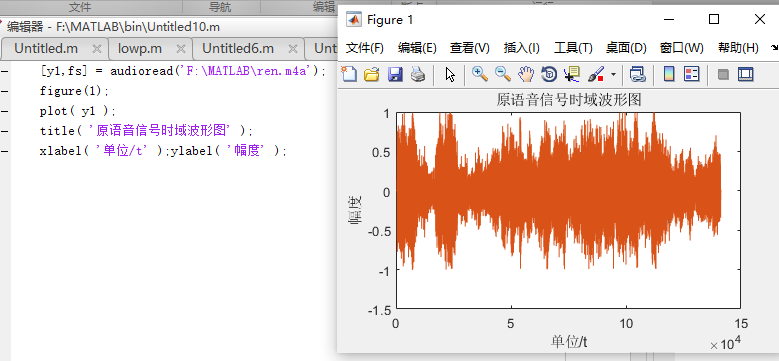2.语音信号频谱分析
首先画出语音信号的时域波形，然后对语音信号进行频谱分析。在matlab中利用fft对信号进行快速傅里叶变换，得到信号的频谱特性。

Matlab的信号处理工具箱中的函数FFT可用于对序列的快速傅里叶变换分析，其调用格式是y=fft(x,N)，其中，x是序列，y是序列的FFT变换结果，N为整数，代表做N点的FFT，若x为向量且长度小于N，则函数将x补零至长度N；若向量x长度大于N，则截断x使之长度为N。

N= length(y1);
f1= (0:N-1)*fs/N;
y2 = fft (y1,N);
plot( f1, abs(y2) );
axis([-1000 5000 0 3000]);
title( '原语音信号频域波形图' );
xlabel('单位');ylabel('幅度');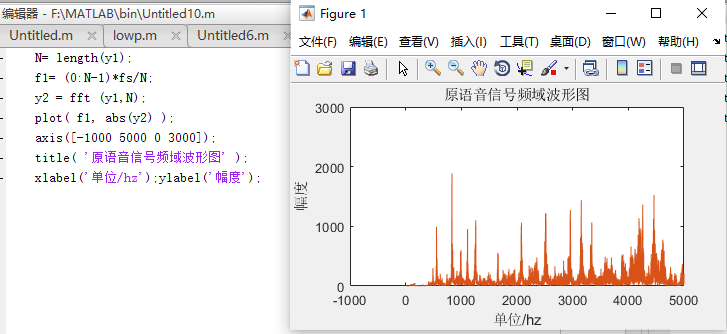原始语音信号的频率基本上集中在5kHz以内。其中原始语音信号FFT频谱和原始语音信号频谱的区别是：前者是频率为1递增的频谱，而后者是以f1= (0:N-1)*fs/N递增，后者是在"不小于原始信号的频率（采样定理）"上完全展开的频谱。

3.设计滤波器和画出频率响应
根据语音信号的特点给出有关滤波器的新能指标：
①	低通滤波器的性能指标：fp=1000Hz，fc=1200Hz，As=100dB，Ap=1dB；

低通数字滤波器的设计采用二次映射的方法，就是先将整个s平面压缩到s1平面的一个
的横形条带范围内，然后再将这个条带映射到z平面上，就能建立s平面到z平面的一一对应关系。映射关系为  ，其中T为抽样周期。

Fp=1000;Fs=1200;Ft=8000;As=100;Ap=1;
wp=2*pi*Fp/Ft;ws=2*pi*Fs/Ft;
[n,wn]=ellipord(wp,ws,Ap,As,'s');
[b,a]=ellip(n,Ap,As,wn,'s');
[B,A]=bilinear(b,a,1);
[h,w]=freqz(B,A);
figure(2);
plot(w*Ft/pi/2,abs(h));
title('低通滤波器');
xlabel('频率');
ylabel('幅度');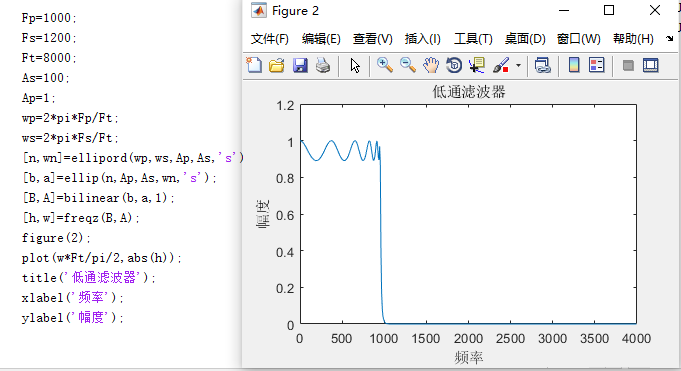②	高通滤波器的性能指标：fp=4500Hz，fc=5000Hz，As=100dB，Ap=1dB；
双线性变换法获得的数字滤波器频率响应特性中不会出现混叠现象，因此可以适用于高通和带通滤波器的设计。IIR数字滤波器的设计通常要借助于模拟低通滤波器的设计，由原型低通滤波器到其他形式IIR数字滤波器的频带变换有模拟频带变换法和数字频带变换法。
Fp=4500;Fs=5000;
As=100;Ap=1;
wp=2*pi*Fp/Ft;
ws=2*pi*Fs/Ft;
[n,wn]=ellipord(wp,ws,Ap,As,'s');
[b,a]=ellip(n,Ap,As,wn,'high','s');
[B,A]=bilinear(b,a,1);
[h,w]=freqz(B,A);
figure(4);
plot(w*Ft/pi/2,abs(h));
title('高通滤波器');
xlabel('频率');
ylabel('幅度');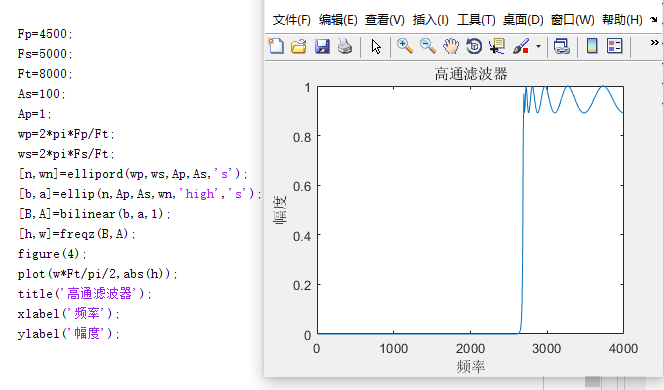③	带通滤波器的性能指标：fp1=1200Hz，fp2=3000hZ，
fc1=1000Hz，fc2=3200Hz，As=100dB，Ap=1dB；
Fp1=1200;Fp2=3000;Fs1=1000;Fs2=3200;
As=100;
Ap=1;
wp=[2*pi*Fp1/Ft,2*pi*Fp2/Ft];
ws=[2*pi*Fs1/Ft,2*pi*Fs2/Ft];
[n,wn]=ellipord(wp,ws,Ap,As,'s');
[b,a]=ellip(n,Ap,As,wn,'s');
[B,A]=bilinear(b,a,1);
[h,w]=freqz(B,A);
figure(6);
plot(w*Ft/pi/2,abs(h));
title('带通滤波器');
xlabel('频率');
ylabel('幅度');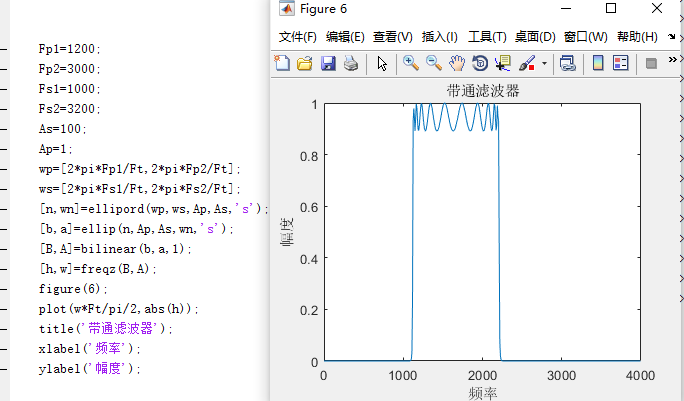4.用滤波器对信号进行滤波
5.滤波前后语音信号的波形及频谱
（1）低通
[x,fs]=audioread('F:\MATLAB\ren.m4a');
x=x(:,1);Y=fft(x);
y=filter(B,A,x);Y1=fft(y);
n=0:length(x)-1;t=(0:FS-1)/fs;
figure(7);
subplot(3,1,1);plot(t,y);grid on;
title('低通滤波器滤波后时域波形图');
xlabel('时间');
ylabel('幅度');
subplot(3,1,2);plot(n,abs(Y));grid on;
title('滤波前语音信号频谱');
xlabel('频率');
ylabel('•幅度');
axis([0 1000000 0 8]);
subplot(3,1,3);plot(n,abs(Y1));grid on;
title('Â滤波后语音信号频谱');
xlabel('频率');
ylabel('•幅度');
axis([0 1000000 0 8]);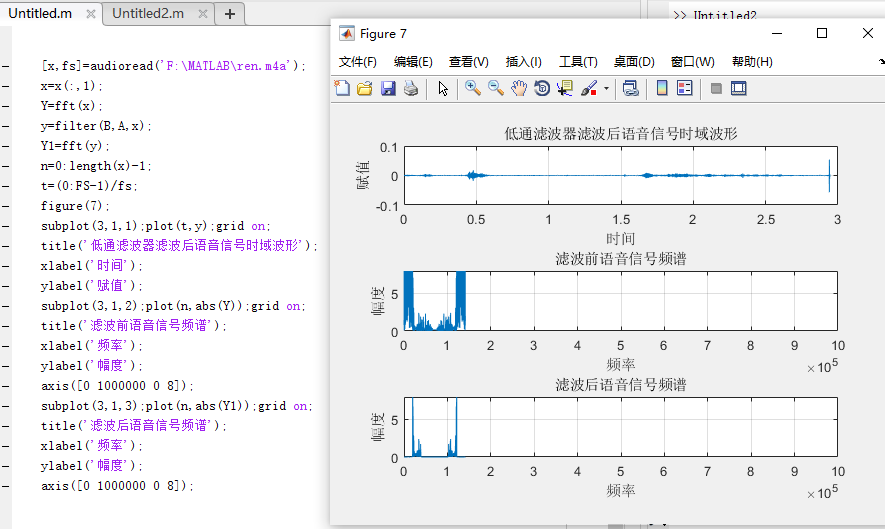（2）高通
[x,fs]=audioread('F:\MATLAB\ren.m4a');
x=x(:,1);
Y=fft(x);
y=filter(B,A,x);
Y1=fft(y);
n=0:length(x)-1;
t=(0:FS-1)/fs;
figure(7);
subplot(3,1,1);plot(t,y);grid on;
title('¸高通滤波器滤波后语音信号时域波形');
xlabel('时间');
ylabel('幅度');
subplot(3,1,2);plot(n,abs(Y));grid on;
title('滤波前语音信号频谱');
xlabel('频率');
ylabel('幅度');
axis([0 1000000 0 8]);
subplot(3,1,3);plot(n,abs(Y1));grid on;
title('滤波后语音信号频谱');
xlabel('频率');
ylabel('幅度');
axis([0 1000000 0 8]);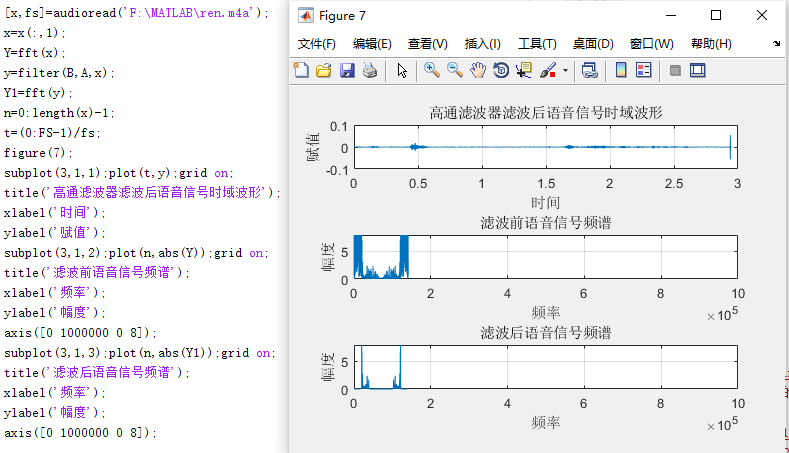（3）带通
[x,fs]=audioread('F:\MATLAB\ren.m4a');
x=x(:,1);
Y=fft(x);
y=filter(B,A,x);
Y1=fft(y);
n=0:length(x)-1;
t=(0:FS-1)/fs;
figure(7);
subplot(3,1,1);plot(t,y);grid on;
title('带通滤波器滤波后语音信号时域波形');
xlabel('时间');
ylabel('¸幅度');
subplot(3,1,2);plot(n,abs(Y));grid on;
title('滤波前语音信号频谱');
xlabel('频率');
ylabel('幅度');
axis([0 1000000 0 5]);
subplot(3,1,3);plot(n,abs(Y1));grid on;
title('滤波后语音信号频谱');
xlabel('频率');
ylabel('幅度');
axis([0 1000000 0 5]);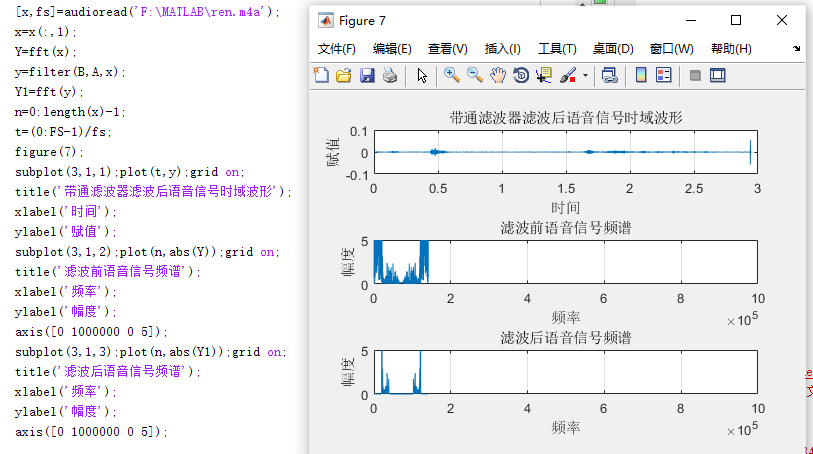低通、高通、带通三种滤波器中，比较后发现，三种滤波器都可以有效的达到滤波效果。其中滤波效果比最好的是低通滤波器，对原始语音影响最小，其他两种滤波效果稍差一些。通过调试可以得知，在设计滤波器中，阶数N越大，滤波器结构越复杂，精度越高，滤波效果越好。

6.回放语音信号
用sound函数播放语音
sound(y1,fs);

听到了一开始Windows录制的音乐
五、实验思考
1、对原始信号作频谱分析时，画出的频谱图关于横轴对称，且成镜像分布，由于使用plot函数画图时没有使用abs(y)来取绝对值，所以频谱的幅值有正有负；而且没有设置横纵坐标轴的范围，所以横轴值过大，频谱成镜像分布。通过查阅plot函数使用格式，修改程序plot(f,abs(y))，添加坐标轴设置函数axis([x1 x2 y1 y2]);grid，解决问题。
2、设计带通滤波器时，下阻带截止频率和通带下截止频率始终会对原始信号造成影响。
调试参数时Rp、As与带阻和低通都有较大差距。将加噪后的语音信号经过带通滤波器，使滤波器滤除原始信号，留下噪声信号，再用加噪信号减去噪声信号可得到原始语音，多次调整fp1和fs1使其到最佳数值，尽量减少对原始噪声的影响。
六、实验收获与总结
通过此次实验，使我对滤波器有了更深的认识，特别是滤波器参数对滤波器性能的影响，在实验中，也遇到了些困难，例如希望可以在界面中播放滤波前后的信号，才知道不同的function是独立执行的，如果想在一个function中使用其他function中变量的值，则需要用save和load来分别保存和调用变量。也认识到matlab软件功能的强大，今后我还需更加努力完善自己，用会，用精matlab来分析解决问题，将理论联系实际，获得更大提高。


展开全文• 画出采样语音信号的时域波形和频谱图；给定滤波器的性能指标，采用窗函数法或双线性变换设计滤波器，并画出 滤波器的频率响应；然后用自己设计的滤波器对采集的信号进行滤波，画出滤波后信号的时域波形和频谱，并...
大三时数字信号处理做过一个简单的语音信号处理。复制过来。课题内容录制一段语音信号，对录制的信号进行采样；画出采样后语音信号的时域波形和频谱图；给定滤波器的性能指标，采用窗函数法或双线性变换设计滤波器，并画出 滤波器的频率响应；然后用自己设计的滤波器对采集的信号进行滤波，画出滤波后信号的时域波形和频谱，并对滤波前后的信号进行对比，分析信号的变化；最后，实现对原始信号进行处理，包括：加噪声、快放、慢放、变声等。 具体实现 1．语音信号的采集 　　利用手机录音机，录制一段话音（我们所录的是---“现在所录的是男声”）。然后在Matlab软件平台下，利用函数audioread对语音信号进行采样。通过audioread函数的使用，我们很快理解了采样频率、采样位数等概念。 2．原始语音信号的频谱分析 　　我们首先画出语音信号的时域波形；然后对语音号进行快速傅里叶变换，得到信号的频谱特性，从而加深对频谱特性的理解。 程序如下： [x,fs]=audioread('nansheng.wav'); %打开语音信号  sound(x,fs); %播放语音信号 N=length(x); %长度 n=0:N-1; w=2*n*pi/N;  y1=fft(x); %对原始信号做FFT变换 subplot(2,1,1);  plot(n,x) %做原始语音信号的时域波形图 title('原始语音信号时域图'); xlabel('时间t'); ylabel('幅值'); subplot(2,1,2); %做原始语音信号的频谱图 plot(w/pi,abs(y1)); title('原始语音信号频谱') xlabel('频率Hz'); ylabel('幅度'); 波形如下：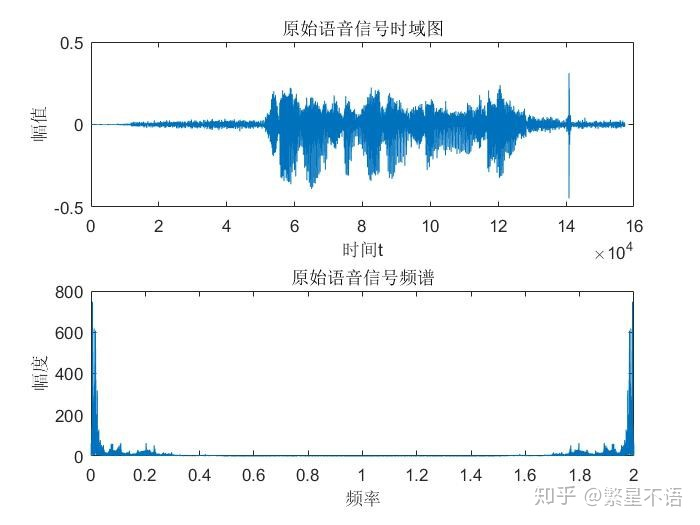3.加噪语音信号并对其FFT频谱分析程序如下：[x,fs]=audioread('mei.wav');n=length(x);x_p=fft(x,n);f=fs*(0:n/2-1)/n;figure(1)subplot(2,1,1); plot(x); title('原始语音信号采样后的时域波形'); xlabel('时间轴')ylabel('幅值A') subplot(2,1,2); plot(f,abs(x_p(1:n/2))); title('原始语音信号采样后的频谱图'); xlabel('频率Hz'); ylabel('频率幅值');noise=0.005*randn(1,n);x_z=x+noise';sound(x_z,fs)n=length(x);x_zp=fft(x_z,n);f=fs*(0:n/2-1)/n;figure(2)subplot(2,1,1);plot(x_z); title('加噪语音信号时域波形');xlabel('时间轴')ylabel('幅值A')subplot(2,1,2); plot(f,abs(x_zp(1:n/2)));title('加噪语音信号频谱图'); xlabel('频率Hz'); ylabel('频率幅值');波形如下：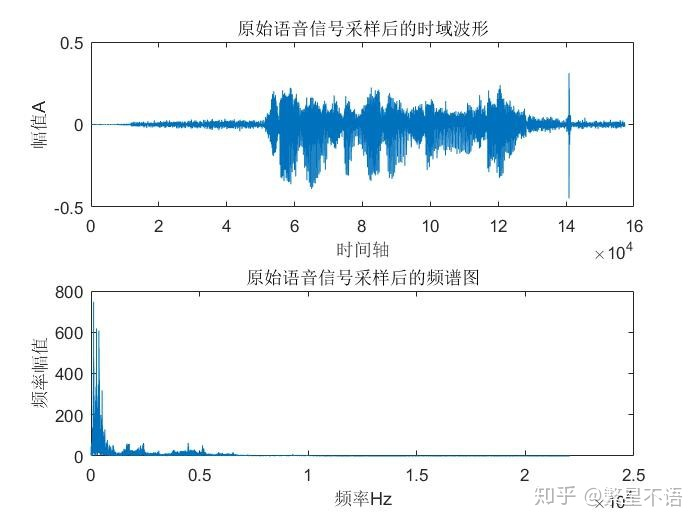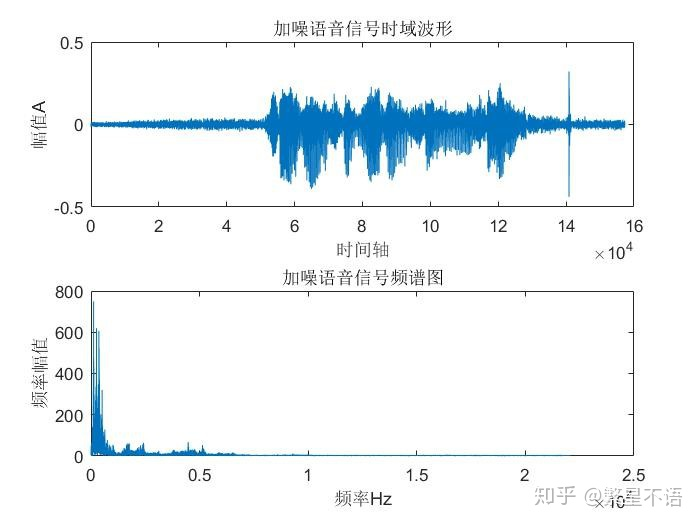4. 滤波器设计我们用双线性变换法设计了一个数字低通滤波器对原始语音信号进行了滤波处理。程序如下：fp=800;fs=1300;rs=35;rp=0.5;Fs=44100; wp=2*Fs*tan(2*pi*fp/(2*Fs));ws=2*Fs*tan(2*pi*fs/(2*Fs)); [n,wn]=buttord(wp,ws,rp,rs,'s'); [b,a]=butter(n,wn,'s'); [num,den]=bilinear(b,a,Fs); [h,w]=freqz(num,den,512,Fs); figure(1) plot(w,abs(h)); xlabel('频率/Hz');ylabel('幅值'); title('巴特沃斯低通滤波器幅度特性'); axis([0,5000,0,1.2]); grid on; [s1,Fs]=audioread('mei.wav'); x1=s1(:,1);sound(x1,Fs); N1=length(x1); Y1=fft(x1,N1); f1=Fs*(0:N1-1)/N1; t1=(0:N1-1)/Fs; figure(2) plot(f1,abs(Y1)) xlabel('频率/Hz');ylabel('幅度');title('原始信号频谱'); grid on;axis([0 50000 0 200]) y=filter(num,den,x1); sound(y,Fs);N2=length(y); Y2=fft(y,N2); f2=Fs*(0:N2-1)/N2; t2=(0:N2-1)/Fs; figure(3) plot(f2,abs(Y2)) xlabel('频率/Hz');ylabel('幅度');title('过滤后信号的频谱'); grid on;axis([0 50000 0 200])波形如下：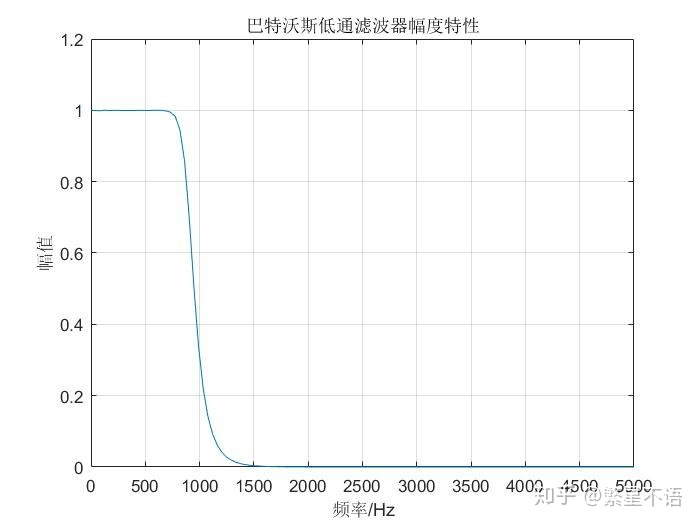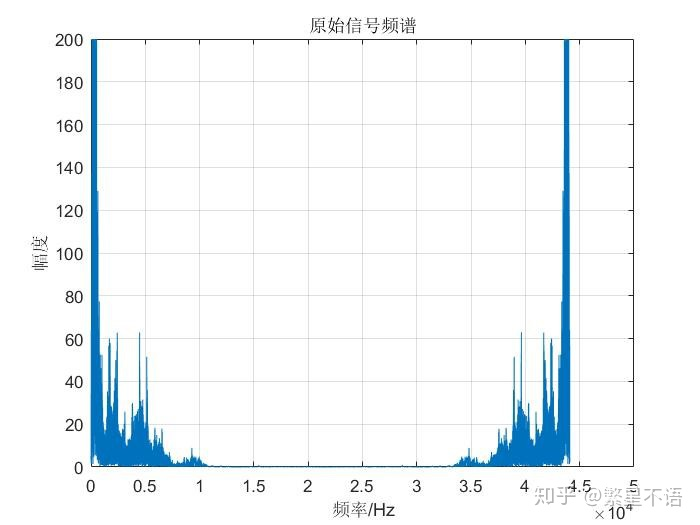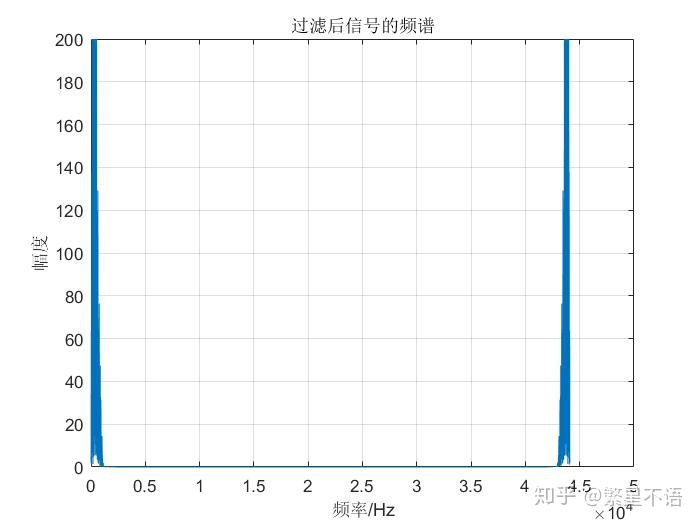5.快放：改变采样频率即可实现。[x,fs]=audioread('nansheng.wav')w=2；M=w*fs; %采样频率加倍6.慢放：[x,fs]=audioread('nansheng.wav')w=0.5；M=w*fs; %采样频率减半7.男女变声处理：[y,fs]=audioread('nansheng.wav'); %读取声音文件x1=y(:,1); %读入的y矩阵有两列,取第1列sound(voice(x1,1.5),fs); N=length(voice(x1,1.5)); %长度n=0:N-1;w=2*n*pi/N; y1=fft(voice(x1,1.5)); %对原始信号做FFT变换subplot(2,1,1); plot(n,voice(x1,1.5)) %做原始语音信号的时域波形图title('变声语音信号时域图');xlabel('时间t');ylabel('幅值');subplot(2,1,2); %做原始语音信号的频谱图plot(w/pi,abs(y1));title('变声语音信号频谱')xlabel('频率');ylabel('幅度');※所调用的Voice函数代码：function Y=voice(x,f) %更改采样率使基频改变 f>1降低;f<1升高f=round(f*1000);d=resample(x,f,1000); %时长整合使语音文件恢复原来时长 W=400; Wov=W/2; Kmax=W*2; Wsim=Wov; xdecim=8; kdecim=2; X=d'; F=f/1000; Ss =W-Wov; xpts = size(X,2);ypts = round(xpts / F); Y = zeros(1, ypts); xfwin = (1:Wov)/(Wov+1); ovix = (1-Wov):0; newix = 1:(W-Wov);simix = (1:xdecim:Wsim) - Wsim; padX = [zeros(1, Wsim), X, zeros(1,Kmax+W-Wov)];Y(1:Wsim) = X(1:Wsim); lastxpos = 0; km = 0; for ypos = Wsim:Ss:(ypts-W)  xpos = round(F * ypos);  kmpred = km + (xpos - lastxpos);  lastxpos = xpos;  if (kmpred <= Kmax)  km = kmpred;  else ysim = Y(ypos + simix);  rxy = zeros(1, Kmax+1);  rxx = zeros(1, Kmax+1);  Kmin = 0;  for k = Kmin:kdecim:Kmax  xsim = padX(Wsim + xpos + k + simix);  rxx(k+1) = norm(xsim);  rxy(k+1) = (ysim * xsim');  end Rxy = (rxx ~= 0).*rxy./(rxx+(rxx==0));  km = min(find(Rxy == max(Rxy))-1);  end xabs = xpos+km; Y(ypos+ovix) = ((1-xfwin).*Y(ypos+ovix)) + (xfwin.*padX(Wsim+xabs+ovix));  Y(ypos+newix) = padX(Wsim+xabs+newix);  endEnd波形如下：f=0.6(主要实现男声变女声）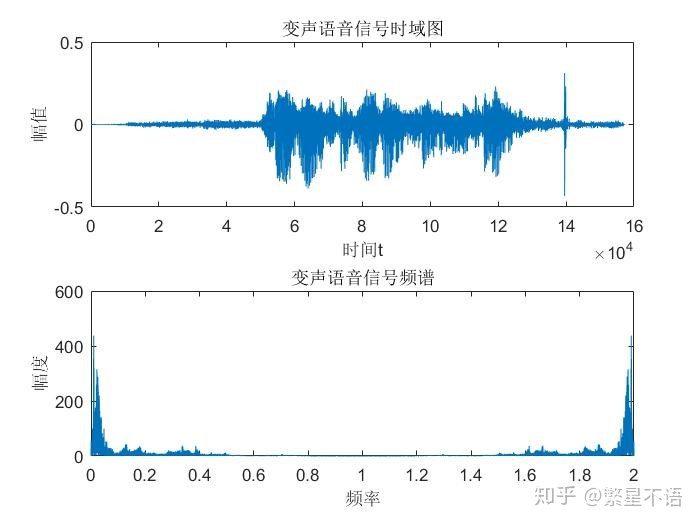f=1.4（实现女声变男声）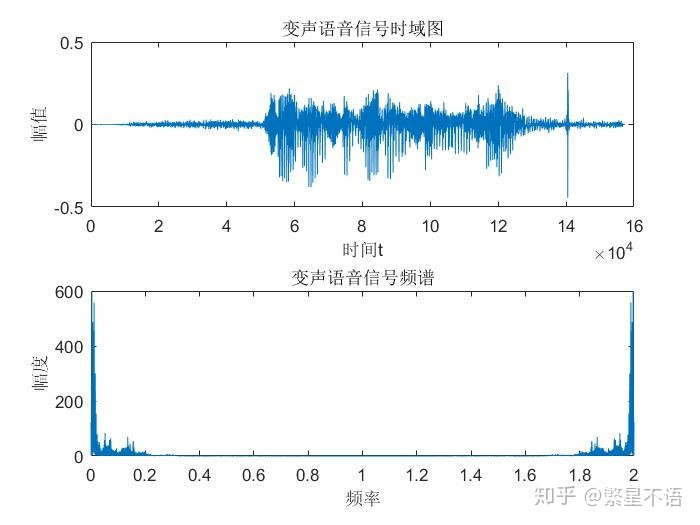展开全文• 基于MATLAB的基本运算语音信号处理课程设计 ，完成语音信号的采集，利用windows自带的录音机或其他软件，录制一段语音，时间在1s以内，并对信号进行采样，画出采样信号的时域频域波形。用窗函数法双线性变换法...
• 一、简介 1．课程设计的目的 通过数字信号处理的课程设计，使学生对信号的采集，处理，传输，...画出采样语音信号的时域波形和频谱图；给定滤波器的性能指标，采用窗函数法和双线性变换设计滤波器，并画出滤波器的频
一、简介
1．课程设计的目的
通过数字信号处理的课程设计，使学生对信号的采集，处理，传输，显示，存储和分析等有一个系统的掌握和理解。巩固和运用数字信号处理课程中的理论知识和实验技能，掌握最基本的数字信号处理的理论和方法，培养学生发现问题，分析问题和解决问题的能力。
2．课程设计的题目
语音信号的采集、分析与处理。
3．设计内容 （主要技术关键的分析、解决思路和方案比较等）
对一段语音信号进行采样；画出采样后语音信号的时域波形和频谱图；给定滤波器的性能指标，采用窗函数法和双线性变换设计滤波器，并画出滤波器的频率响应；然后用自己设计的滤波器对采集的信号进行滤波，画出滤波后信号的时域波形和频谱，并对滤波前后的信号进行对比，分析信号的变化；回放语音信号；最后，设计一个信号处理系统界面。
设计内容：采样一段语音信号；画出语音信号的时域波形和频谱图；给定滤波器的性能指标，设计数字滤波器，并画出滤波器的频率响应；然后用设计的滤波器对采集的信号进行滤波，画出滤波后信号的时域波形和频谱。
关键技术：频谱图的理解；设计数字滤波器；数字滤波的方法；
解决思路：对语音号进行快速傅里叶变换，得到信号的频谱特性；在MATLAB环境中可以利用函数fir设计FIR滤波器，可以利用函数butter设计IIR滤波器；利用MATLAB中的函数freqz画出各滤波器的频率响应。
4、设计原理及步骤
原理：
4.1 语音信号的采集
语音信号是一种模拟信号，首先须经过采样将其转换为数字信号，实质是把连续信号变为脉冲或数字序列。  我们可以用录音软件先录一段wav格式的音频。然后用matlab的audioread函数采集，记住采样频率和采样点。然后用sound函数来使用。
4.2 语音信号的频谱特性
在MATLAB中，可以利用函数fft对信号进行快速傅里叶变换，得到信号的频谱特性。
快速傅氏变换（FFT），是离散傅氏变换的快速算法，它是根据离散傅氏变换的奇、偶、虚、实等特性，对离散傅立叶变换的算法进行改进获得的。
4.3 语音信号的时域分析
语音信号的时域分析就是分析和提取语音信号的时域参数。进行语  音分析时，最先接触到并且也是最直观的是它的时域波形。语音信号本身就是时域信号，因而时域分析是最早使用，也是应用最广泛的一种分析方法，这种方法直接利用语音信号的时域波形。时域分析通常用于最基本的参数分析及应用，如语音的分割、预处理、大分类等。这种分析方法的特点是：
①表示语音信号比较直观、物理意义明确。
②实现起来比较简单、运算且少。
③可以得到语音的一些重要的参数。
④只使用示波器等通用设备，使用较为简单等。
语音信号的时域参数有短时能量、短时过零率、短时白相关函数和短时平均幅度差函数等，这是语音信号的一组最基本的短时参数，在各种语音信号数字处理技术中都要应用。在计算这些参数时使用的一般是方窗或汉明窗。对语音信号进行分析，发现发浊音时，尽管声道有若干个共振峰，但由于声门波引起谱的高频跌落，所以其话音能量约集中在3kHz以下。而发清音时，多数能量出现在较高频率上。高频就意味着高的平均过零率，低频意味着低的平均过零率，所以可以认为浊音时具有较低的过零率，而清音时具有较高的过零率。当然，这种高低仅是相对而言，并没方精确的数值关系。
4.4 语音信号的频域分析
语音信号的频域分析就是分析语音信号的频域持征。从广义上讲，语音信号的频域分析包括语音信号的频谱、功率谱、倒频谱、频谱包络分析等，而常用的频域分析方法有带通滤波器组法、傅里叶变换法、线件预测法等几种。因为语音波是一个非平稳过程，因此适用于周期、瞬变或平稳随机信号的标准傅里叶变换不能用来直接表示语音信号，而应该用短时傅里叶变换对语音信号的频谱进行分析，相应的频谱称为“短时谱”。
4.5 采样定理
在进行模拟与数字信号的转换过程中，当采样大于最高频率的2倍时，则采样之后的数字信号完整的保留了原始信号中的信息，一般实际应用中保证采样频率为信号最高频率的5~10倍。
4.6 采样频率
采样频率是指计算机每秒钟采样多少个声音样本，是描述声音文件的音质、音频、衡量声卡、声音文件的质量标准。采样频率越高，即采样的时间间隔越短，则在单位时间内计算机得到的声音样本数据越多，对声音波形的表示也越准确。
4.7 采样位数
采样位数即采样值或取样值,用来衡量声音波动变化的参数,是指声卡在采集和播放声音文件时所使用数字声音信号的二进制位数。采样频率是指录音设备在一秒钟内对声音信号的采样次数,采样频率越高声音的还原就越真实越自然。采样位数和采样率对于音频接口来说是最为重要的两个指标。无论采样频率如何,理论上来说采样的位数决定了音频数据最大的力度范围。采样位数越多则捕捉到的信号越精确。
二、源代码
function varargout = shengyincaiji(varargin)
% SHENGYINCAIJI M-file for shengyincaiji.fig
%      SHENGYINCAIJI, by itself, creates a new SHENGYINCAIJI or raises the existing
%      singleton*.
%
%      H = SHENGYINCAIJI returns the handle to a new SHENGYINCAIJI or the handle to
%      the existing singleton*.
%
%      SHENGYINCAIJI('CALLBACK',hObject,eventData,handles,...) calls the local
%      function named CALLBACK in SHENGYINCAIJI.M with the given input arguments.
%
%      SHENGYINCAIJI('Property','Value',...) creates a new SHENGYINCAIJI or raises the
%      existing singleton*.  Starting from the left, property value pairs are
%      applied to the GUI before shengyincaiji_OpeningFunction gets called.  An
%      unrecognized property name or invalid value makes property application
%      stop.  All inputs are passed to shengyincaiji_OpeningFcn via varargin.
%
%      *See GUI Options on GUIDE's Tools menu.  Choose "GUI allows only one
%      instance to run (singleton)".
%
% See also: GUIDE, GUIDATA, GUIHANDLES

% Edit the above text to modify the response to help shengyincaiji

% Last Modified by GUIDE v2.5 10-May-2010 15:08:25

% Begin initialization code - DO NOT EDIT
gui_Singleton = 1;
gui_State = struct('gui_Name',       mfilename, ...
'gui_Singleton',  gui_Singleton, ...
'gui_OpeningFcn', @shengyincaiji_OpeningFcn, ...
'gui_OutputFcn',  @shengyincaiji_OutputFcn, ...
'gui_LayoutFcn',  [] , ...
'gui_Callback',   []);
if nargin & isstr(varargin{1})
gui_State.gui_Callback = str2func(varargin{1});
end

if nargout
[varargout{1:nargout}] = gui_mainfcn(gui_State, varargin{:});
else
gui_mainfcn(gui_State, varargin{:});
end
% End initialization code - DO NOT EDIT

% --- Executes just before shengyincaiji is made visible.
function shengyincaiji_OpeningFcn(hObject, eventdata, handles, varargin)
% This function has no output args, see OutputFcn.
% hObject    handle to figure
% eventdata  reserved - to be defined in a future version of MATLAB
% handles    structure with handles and user data (see GUIDATA)
% varargin   command line arguments to shengyincaiji (see VARARGIN)

% Choose default command line output for shengyincaiji
handles.output = hObject;

% Update handles structure
guidata(hObject, handles);

% UIWAIT makes shengyincaiji wait for user response (see UIRESUME)
% uiwait(handles.figure1);

% --- Outputs from this function are returned to the command line.
function varargout = shengyincaiji_OutputFcn(hObject, eventdata, handles)
% varargout  cell array for returning output args (see VARARGOUT);
% hObject    handle to figure
% eventdata  reserved - to be defined in a future version of MATLAB
% handles    structure with handles and user data (see GUIDATA)

% Get default command line output from handles structure
varargout{1} = handles.output;

% --- Executes during object creation, after setting all properties.
function popupmenu2_CreateFcn(hObject, eventdata, handles)
% hObject    handle to popupmenu2 (see GCBO)
% eventdata  reserved - to be defined in a future version of MATLAB
% handles    empty - handles not created until after all CreateFcns called

% Hint: popupmenu controls usually have a white background on Windows.
%       See ISPC and COMPUTER.
if ispc
set(hObject,'BackgroundColor','white');
else
set(hObject,'BackgroundColor',get(0,'defaultUicontrolBackgroundColor'));
end

三、运行结果四、备注
完整代码或者代写添加QQ 1564658423
往期回顾>>>>>>
【特征提取】基于matlab小波变换的音频水印嵌入提取【含Matlab源码 053期】
【语音处理】基于matlab GUI语音信号处理【含Matlab源码 290期】


展开全文• 2.画出采样后的语音信号的时域波形和频谱图； 3.给定滤波器的性能指标，采用窗函数法和双线性变换法设计滤波器， 并划出滤波器的频域响应； 4.用该滤波器对采集的信号进行滤波，画出滤波后信号的时域波形和频谱， 并...
• 画出采样语音信号的时域波形和频谱图；给定滤波器的性能指标，采用窗函数法或双线性变换设计滤波器，并画出 滤波器的频率响应；然后用自己设计的滤波器对采集的信号进行滤波，画出滤波后信号的时域波形和频谱，并...
• 一、简介 1．课程设计的目的 通过数字信号处理的课程设计，使学生对信号的采集，处理，传输，...画出采样语音信号的时域波形和频谱图；给定滤波器的性能指标，采用窗函数法和双线性变换设计滤波器，并画出滤波器的频
• ﻿﻿ 设计要求： ...2)对语音信号进行采集，画出采集后的语音信号的时域波形和频谱图。 理论依据: 1)采样频率：采样频率（也称为采样速度或者采样率）定义了每秒从连续信号 中提取并组
• 1录制一段自己的语音信号并对录制的信号进行采样 2画出采样语音信号的时域波形和频谱图 3通过给定滤波器的性能指标设计数字滤波器并画 出滤波器的频率响应 4用自己设计的滤波器对采集的语音信号进行滤波画 出滤波...
• 一般情况下，极点的个数在12－16个之间就可以足够清晰地描述语音信号的特征了。 语音信号经过预处理，它的每个样值均可由过去若干个样值的线性组合来逼近，同时可以采用使实际语音抽样与线性预测抽样之间的均方差...
• 还蛮用用的语音信号的采集 利用Windows下的录音机或其他软件，录制一段自己的语音，时间控制在1s左右；然后在MATLAB软件平台下，利用函数wavread对语音信号... 2 语音信号的频谱分析 要求首先画出语音信号的时域波形
• Matlab中，可以利用函数FFT对信号进行快速傅立叶变换，得到信号的频谱特性，要求学生首先画出语音信号的时域波形，然后对语音信号进行频谱分析。 3、对语音信号分别加入正弦噪声高斯白噪声，使信噪比为（学号）...
• 画出采样语音信号的时域波形和频谱图；给定滤波器的性能指标，采用窗函数法和双线性变换设计滤波器，并画出滤波器的频率响应；然后用自己设计的滤波器对采集的信号进行滤波，画出滤波后信号的时域波形和频谱，并对...
• 在抽样信号的基础上，通过采样后的的信号与原信号实现一次及多次延迟、叠加产生回波信号，再使用Matlab绘出有回声及无回声语音信号的时域波形和频谱图。再分别用频率抽样法设计的FIR滤波器和冲激相应不变法设计设计...# matlab语音信号的采样和频谱分析matlab 订阅